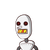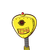# the marked price of a bicycle is rs 2500 and it was sold with discount for rs 2000 find the percentage of discount. std 8 maths ss

the marked price of a bicycle is rs 2500 and it was sold with discount for rs 2000 find the percentage of discount. std 8 maths ssc board chp discount and commission​

### 2 thoughts on “the marked price of a bicycle is rs 2500 and it was sold with discount for rs 2000 find the percentage of discount. std 8 maths ss”

1.cp of cycle= 2500

sp of cycle=2000

difference between cp and sp = 2500-2000

= 500

percentage = 500*100/2500

% = 20%

2.